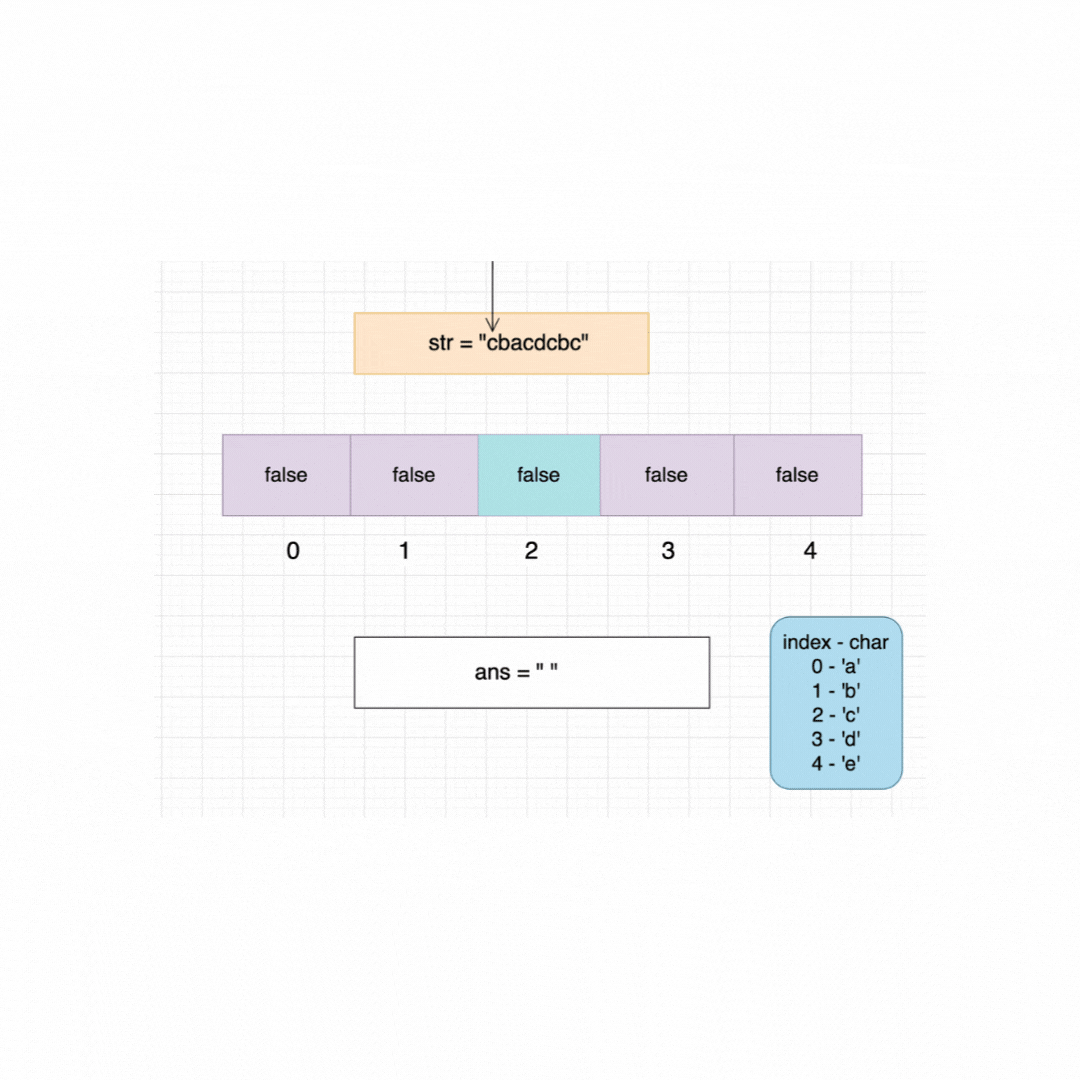# Remove All Duplicates from a String

Problem Statement: Given a String remove all the duplicate characters from the given String.

Examples:

```Example 1:
Input: s = "bcabc"
Output: “bca"

Explanation: Duplicate Characters are removed
Example 2:
Input: s = "cbacdcbc"
Explanation: Duplicate Characters are removed```

Solution:

Disclaimer: Don’t jump directly to the solution, try it out yourself first.

Solution 1: Brute Force

Keep two pointers I, j.

i – > For traverse through the string

j – > to check if the character is already present on the left side of the string.

Traverse through the string and for every index i check if str[i] is already present on the left side of the curr idx by looping through (j —> 0 – i -1).

if the same character is found, break through the loop. Now if(i == j) which means we haven’t found the same character add it to the res string. At any point, if the same character is found then i and j will not be the same.

Code:

## C++ Code

``````#include<bits/stdc++.h>

using namespace std;

string removeDuplicateLetters(string s) {
string ans = "";
for (int i = 0; i < s.length(); i++) {
int j = 0;
for (j = 0; j < i; j++) {
if (s[i] == s[j]) //same character found
{
break;
}
}
if (i == j) {
ans += s[i];
}
}
return ans;
}
int main() {
string str = "cbacdcbc";
cout<<"Original String: "<<str<<endl;
cout <<"After removing duplicates: " <<removeDuplicateLetters(str) << endl;
return 0;
}
``````

Output:

Original String: cbacdcbc
After removing duplicates: cbad

Time Complexity: O(N^2)

Space Complexity: O(1)

## Java Code

``````import java.util.*;

class Main {
public static String removeDuplicateLetters(String s) {

String ans = "";
for (int i = 0; i < s.length(); i++) {
int j = 0;
for (j = 0; j < i; j++) {
if (s.charAt(i) == s.charAt(j)) //same character found
{
break;
}
}
if (i == j) {
ans += s.charAt(i);
}
}
return ans;
}
public static void main(String[] args) {
String str = "cbacdcbc";
System.out.println("Original String: "+str);
System.out.println("After removing duplicates: "+removeDuplicateLetters(str));

}
}
``````

Output:

Original String: cbacdcbc
After removing duplicates: cbad

Time Complexity: O(N^2)

Space Complexity: O(1)

Solution 2: Using a boolean array

The input string will only contain lowercase alphabets. So let’s create a boolean array of size 26 initialized to false.

Consider the index as the ASCII value of the character.

For example: for character ch = ‘c’. The index value will be 2.this can be achieved by doing s[i] – ‘a’ => 99 – 97 = 2 (Since ascii value of ‘c’ = 99 and ‘a’ = 97).

Keep a pointer i at the starting of the string. check if the character is already visited or not. if(s[i] – ‘a’) is false then add that character to ans and make it true.

Repeat it till we reach the end of the string.

Dry Run:

For the dry run let’s consider the size of the boolean map to be 5.Code:

## C++ Code

``````#include<bits/stdc++.h>

using namespace std;

string removeDuplicateLetters(string s) {
string ans = "";
vector < bool > map(26, false);
for (int i = 0; i < s.length(); i++) {
if (map[s[i] - 'a'] == false) {
ans += s[i];
map[s[i] - 'a'] = true;
}
}
return ans;
}
int main() {
string str = "cbacdcbc";
cout << "Original String: "<<str<<endl<<"After removing duplicates: "
<<removeDuplicateLetters(str) << endl;
return 0;
}
``````

Output:

Original String: cbacdcbc
After removing duplicates: cbad

Time Complexity: O(N)

Space Complexity: O(1)

## Java Code

``````import java.util.*;

class Main {
public static String removeDuplicateLetters(String s) {

String ans = "";
boolean[] map = new boolean;
for (int i = 0; i < s.length(); i++) {
if (map[s.charAt(i) - 'a'] == false) {
ans += s.charAt(i);
map[s.charAt(i) - 'a'] = true;
}
}
return ans;
}
public static void main(String[] args) {
String str = "cbacdcbc";
System.out.println("Original String: "+str+"\nAfter removing duplicates:
"+removeDuplicateLetters(str));

}
}
``````

Output:

Original String: cbacdcbc
After removing duplicates: cbad

Time Complexity: O(N)

Space Complexity: O(1)

Special thanks to P.C.Bhuvaneshwari for contributing to this article on takeUforward. If you also wish to share your knowledge with the takeUforward fam, please check out this article